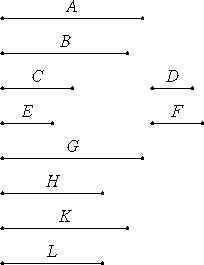# Proposition 5

Plane numbers have to one another the ratio compounded of the ratios of their sides.

Let A and B be plane numbers, and let the numbers C and D be the sides of A, and E and F the sides of B.I say that A has to B the ratio compounded of the ratios of the sides.

VIII.4

The ratios being given which C has to E and D to F, take the least numbers G, H, and K that are continuously in the ratios C, E, D, and F, so that C is to E as G is to H, and D is to F as H is to K.

Multiply D by E to make L.

VII.17

Now, since D multiplied by C makes A, and multiplied by E makes L, therefore C is to E as A is to L. But C is to E as G is to H, therefore G is to H as A is to L.

VII.17

Again, since E multiplied by D makes L, and further multiplied by F makes B, therefore D is to F as L is to B. But D is to F as H is to K, therefore H is to K as L is to B.

VII.14

But it was also proved that, H as G is to H as A is to L, therefore, ex aequali, L as G is to K as A is to B.

But G has to K the ratio compounded of the ratios of the sides, therefore A also has to B the ratio compounded of the ratios of the sides.

Therefore, plane numbers have to one another the ratio compounded of the ratios of their sides.

Q.E.D.

## Guide

#### Compounded ratios

Compound ratios such as these only appear in a few places in the Elements. They appear here in this proposition, and in VI.23, an analogous proposition for rectangles and parallelograms. But duplicate and triplicate ratios are also special kinds of compound ratios, and they are used in Books VI, VIII, IX, X, XI, and XII. Duplicate and triplicate ratios were defined in general in V.9-10, where they are defined as the ratio of the ends of a continued proportion (with constant ratio). That is, if a : b = b : c, then the duplicate ratio of a : b is a : c. For lines, the construction of the third proportional c needed to duplicate the ratio a : b was done in proposition VI.11, but for numbers, the third proportional can be constructed by VIII.2.

The ratio compounded from two given ratios a : b and b : c is just the ratio a : c. But if the middle term b is not shared by the two given ratios, then equal ratios must be found that do have a shared middle term.

To find the ratio compounded from two given ratios a : b and c : d, first find e, f, and g so that e : f = a : b and f : g = c : d. Then, the ratio compounded from the ratios a : b and c : d will be the same as the ratio compounded from the ratios e : f and f : g, namely e : g. For numbers, this construction was done in the previous proposition VIII.4.

#### This proposition

The problem is to find a ratio compounded from two ratios c : e and d : f. Since

c : e = cd : de,
and
d : f = de : ef,

therefore, the ratio of the plane numbers cd : ef will do.

Euclid’s proof is complicated because (1) his symbolic notation is limited, and (2) the application of VIII.4 to find the least numbers continuously in the ratios c : d and e : f makes the proof more difficult.

#### Outline of the proof

Let the plane number a be the product cd of its sides, and let the plane number b be the product ef of its sides. Use VIII.4 to construct a continued ratio g : h : k so that g : h = c : e and h : k = d : f so that g : k is the ratio compounded of the ratios c : e and d : f of the sides.

Since a = cd, therefore c : e = a : de, and so g : h = a : de. Since b = ef, therefore d : f = de : b, and so h : k = de : b. From the two proportions g : h = a : de and h : k = de : b therefore, ex aequali, g : k = a : b. Thus, ratio the plane numbers is the ratio compounded of the ratios of their sides.